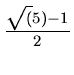Next: 3.12.2 Skip Lists: Experimental Analysis Up: 3.12 Programming Assignments Previous: 3.12 Programming Assignments

## 3.12.1 Hashing: Experimental Analysis

The objective of this assignment is to compare the performance of different hash table organizations, hashing methods, and collision resolution techniques.

Generation of Keys

Assume that your keys are character strings of length 10 obtained by scanning a C program. Call these as tokens. Define a token as any string that appears between successive occurrences of a forbidden character, where the forbidden set of characters is given by:

F = {!,",#,%,&,(,),*, + , - ,.,/, : ,;, < , > , = ,?,[,],{,}, ,|, backslash, comma, space}

Exceptions to the above rule are:
• Ignore anything that appears as comment (that is between /* and */)
• Ignore anything that appears between double quotes
That is, in the above two cases, the character strings are not to be taken as tokens. If a string has less than 10 characters, make it up to 10 characters by including an appropriate number of trailing *'s. On the other hand, if the current string has more than 10 characters, truncate it to have the first ten characters only.

From the individual character strings (from now on called as tokens), generate a positive integer (from now on called as keys) by summing up the ASCII values of the characters in the particular token. Use this integer key in the hashing functions. However, remember that the original token is a character string and this is the one to be stored in the hash table.

Hash Table Methods to be Evaluated

As discussed in the class, the following eleven schemes are to be evaluated.

1.
Open with division method for hashing and unsorted lists for chaining
2.
Open with division method for hashing and sorted lists for chaining
3.
Open with multiplication method for hashing and unsorted lists for chaining
4.
Open with multiplication method for hashing and sorted lists for chaining
5.
Closed with simple coalesced hashing
6.
Closed with linear probing
7.
8.
Closed with double hashing
9.
Closed with linear probing and Knuth-Amble method (Keys with same hash value appear in descending order)
10.
Closed with quadratic probing and Knuth-Amble method
11.
Closed with double hashing and Knuth-Amble method

Hashing Functions

For the sake of uniformity, use the following hashing functions only. In the following, m is the hash table size (that is, the possible hash values are, 0, 1,..., m - 1), and x is an integer key.

1.
Division Method

h(x) = x   mod   m

2.
Multiplication Method

h(x) = Floor(m*Fraction(k*x))

where k =, the Golden Ratio.
3.
Linear Probing

h(x, i) = (h(x, 0) + i)   mod   m;i = 0,..., m - 1

4.

h(x, i) = (h(x, 0) + i + i2)   mod   m;i = 0,..., m - 1

5.
Double Hashing
Use the division method for h(x) and the multiplication method for h'(x).

Inputs to the Program

The possible inputs to the program are:

• m: Hash table size. Several values could be given here and the experiments are to be repeated for all values specified. If nothing is specified, assume all primary numbers between 7 and 97.
• n: The initial number of insertions to be made for setting up a hash table with a particular load factor.
• M: This is a subset of the set {1, 2,..., 11} indicating the set of methods to be investigated.
• I: This is a decimal number from which a ternary string is to be generated (namely the radix-3 representation of I). In this representation, assume that a 0 represents the search operation, a 1 represents the insert operation, and a 2 represents the delete operation.
• A C program, from which the tokens are to be picked up for setting up and experimenting with the hash table.

What should the Program Do?

1.
For each element of M and for each value of m, do the following.
2.
Scan the given C program and as you scan, insert the first n tokens scanned into an initially empty hash table.
3.
Now scan the rest of the C program token by token, searching for it or inserting it or deleting it, as dictated by the radix-3 representation of I. Note that the most significant bit (tigit?) is to be considered first while doing this and you proceed from left to right in the radix-3 representation. For each individual operation, keep track of the number of probes.
4.
Compute the average number of probes for a typical successful search, unsuccessful search, insert, and delete.
5.
Repeat Steps 2, 3, and 4 for each value of M and each value of m.
6.
For each individual method investigated, obtain a table that lists the average number of probes for the four cases for various values of m. The table entries can be of the following format:
 Hash table size Average Number of Probes for Usearch Ssearch Insert Delete
7.
For each individual value of m, obtain a table that lists the average number of probes for various methods. The table entries can be of the following format:
 Hash table method Average Number of Probes for Usearch Ssearch Insert DeleteNext: 3.12.2 Skip Lists: Experimental Analysis Up: 3.12 Programming Assignments Previous: 3.12 Programming Assignments
eEL,CSA_Dept,IISc,Bangalore